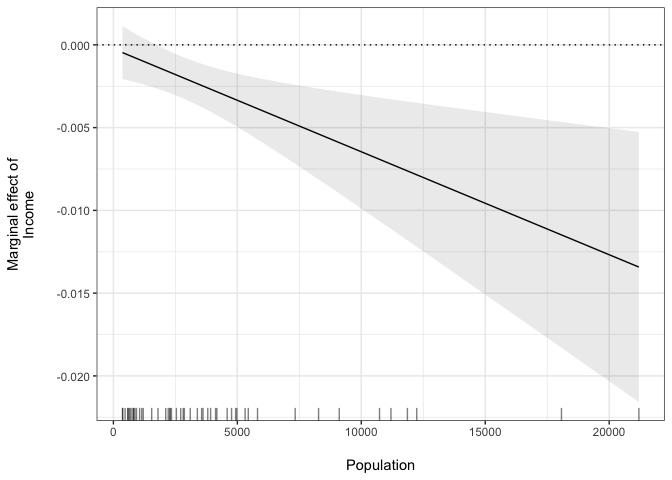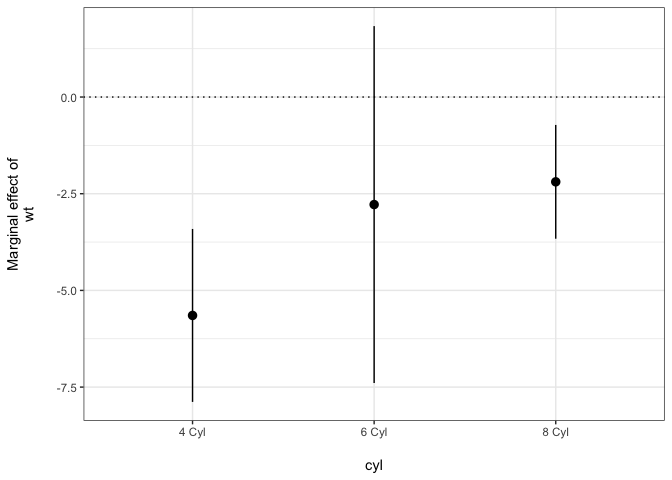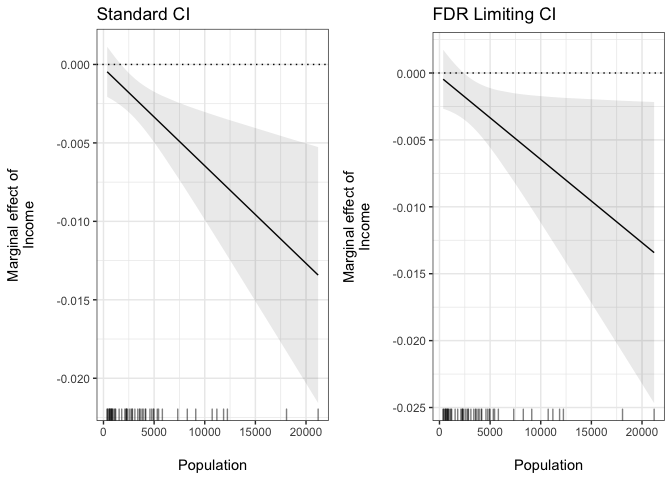Plot Marginal Effects from Linear Models

Plot marginal effects for interactions estimated from linear models.

A simple R package to plot marginal effects from interactions estimated from linear models.

Examples

Continuous Term 2

The package contains one simply function: plot_me for plotting marginal effects from interactions estimated from models estimated with the lm function in base R. For example, when the second term is continuous:## Categorical (Factor) Term 2

When the second term in the interaction is a categorical (factor) variable then point-ranges are plotted. Note that the marginal effect is in terms of the reference category:Note that point ranges will also be used if there are five or fewer fitted values.

Determining the False Positive Rate

Esarey and Sumner show that pointwise confidence intervals from marginal effect plots produce statistically significant findings at a rate that can be larger or smaller than is warrented. plot_me allows users to specify ci_type = 'fdr' to find confidence intervals that correct for overly confident marginal effects in the face of multiple comparisons. FDR stands for “False Discovery Rate”. For example:

Here is the result compared with standard confidence intervals:

## t-statistic used: 2.269You can also use the t_statistic argument to supply custom t-statistics for creating the marginal effect confidence intervals. This is useful if you want to use a funciton like findMultiLims from the interactTest package to find t-statistics that can be used to correct confidence intervals for underconfidence.

The interplot package also has some of the same capabilities as plotMElm.

Version 0.1.6

• Change README figure files paths for CRAN.

Version 0.1.5

• Resolved a bug where plot_me didn't actually check term1's type.

Version 0.1.4

• Allow user to find false discovery rate limiting confidence intervals with the argument ci_type.

• Allow the user to specify custom t-statistics for finding the confidence intervals.

Version 0.1.3

• Correctly find and plot marginal effects with Term 2 is a categorical (factor) variable.

Version 0.1.2

• Use point range when Term 2 has less than or equal to 5 values.

Version 0.1.1

• Allow any confidence level with ci argument.

• Return a data frame instead of a plot with plot argument.

Thanks to Vincent Arel-Bundock for both contributions.

Reference manual

install.packages("plotMElm")

0.1.5 by Christopher Gandrud, 4 years ago

Report a bug at https://github.com/christophergandrud/plotMElm/issues

Browse source code at https://github.com/cran/plotMElm

Authors: Christopher Gandrud [aut, cre]

Documentation:   PDF Manual